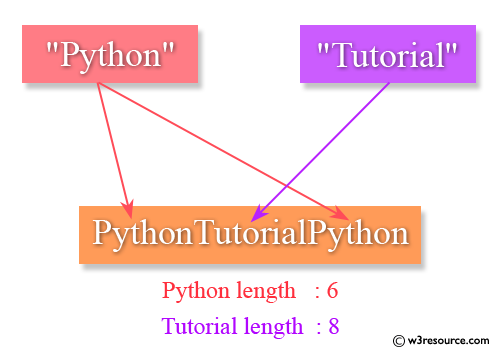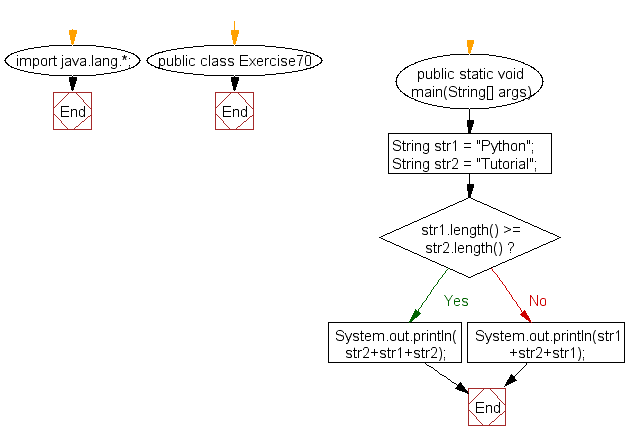﻿ Java exercises: Create a string in the form short_string + long_string + short_string from two strings - w3resource# Java Exercises: Create a string in the form short_string + long_string + short_string from two strings

## Java Basic: Exercise-70 with Solution

Write a Java program to create a string in the form short_string + long_string + short_string from two strings. The strings must not have the same length.

Pictorial Presentation:Sample Solution:

Java Code:

``````import java.lang.*;
public class Exercise70 {
public static void main(String[] args)
{
String str1 = "Python";
String str2 = "Tutorial";

if(str1.length() >= str2.length())
System.out.println( str2+str1+str2);
else
System.out.println(str1+str2+str1);
}
}
```
```

Sample Output:

```PythonTutorialPython
```

Flowchart:Java Code Editor:

What is the difficulty level of this exercise?

﻿

## Java: Tips of the Day

initial:

Returns all the elements of an array except the last one. Use Arrays.copyOfRange() to return all except the last one

```public static <T> T[] initial(T[] elements) {
return Arrays.copyOfRange(elements, 0, elements.length - 1);
}
```

Ref: https://bit.ly/3zEKw9u